High School Graduation Requirements: Math - 2.5 creditsHome PHOTO GALLERY COLLEGE PREP AWARDS & ACHIEVEMENTS ENTREPRENEURS EXAM SCORES LYRICS TO OUR CD Rachel - Background Info English - 4 cr. Math - 2.5 credits Science - 3.5 cr. Social Studies - 3 Health & Fitness 2 Arts - 2 credits Occupational Education - 1.5 Electives H.S. TRANSCRIPT Advanced Placement Volunteer Work Sports PRACTICE TESTS COLLEGE-PREP ENGLISH OUR MUSIC VIDEOALGEBRA                                          1  Credit
GEOMETRY                                        1  Credit
3D MATH                                             .5 Credit

2.5 Credits

Summer 2007

Statistics  (registering for Advanced Placement)
Pre-Calculus

ALGEBRA is a language of puzzle pieces, which can help us visualize and understand things that we can't understand with arithmetic or basic math.  The puzzle pieces include rational, irrational, and real numbers, variables, expressions, terms, coefficients, and constants.

Quick Review:

Real numbers have real-world values like dollar amounts, weights, distances, age, temperature, and so on.

Rational numbers are real integers and fractions, and relate to the "ratio".  Rational numbers can be written as a ratio, or quotient, of two integers.

Ex:  The fraction ½ is the ratio of 1 to 2.

Ex:  Three can be expressed as 3/1, which is a ratio of 3:1.

Ex:  Since 0.57 can be written as the fraction 57/100, it is a rational number.

Irrational numbers are real numbers that can't be expressed as a quotient of two integers, such as non-repeating and non-terminating numbers.  To calculate the area of a circle, a non-repeating, non-terminating irrational number is used, which has a symbol all it's own:Coefficients are the number alongside the variables (which are letters that represent mysterious numberous).  In 3x2 + 2y + 7xy + 5, the 3 is the coefficient of the first term. The 2 is the coefficient of the second term, and the 7 is the coefficient of the third term.  The 5 is the *constant*, because it doesn't have a mysterious *variable* that can change the value.

Equations are mathematical expressions that contain an equals sign.

Inequality symbols are used when you don't know what the expression equals:

> greater than
< less than
< less than or equal to
> greater than or equal to

Properties of numbers are commutative (addition and multiplication can be done in any order), associative (addition and multiplication can be done in any choice of groupings), and distributive (terms multiplied by terms in parenthesis, must be "distributed" across all the terms inside the parenthesis:

2x(5 + y) = 10x + 2xy

Density (decimal numbers can be made more exact), and identity (zero added to any number equals the number).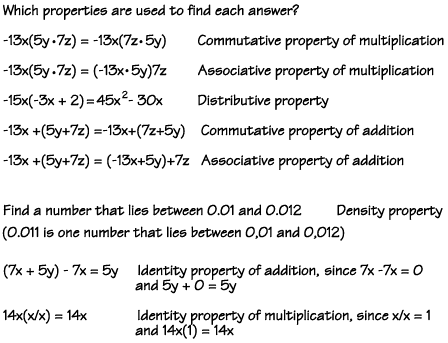Exponents: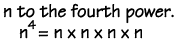Any number raised to the zero power (except 0) equals 1.

Any number raised to the power of one equals itself.

To multiply terms with the same base, add the exponents.

To divide terms with the same base, subtract the exponents.

When a product has an exponent, each factor is raised to that power.

A number with a negative exponent equals its reciprocal with a positive exponent.

Rules of 1 - Any number raised to the power of "one" equals itself. This makes sense, because the power shows how many times the base is multiplied by itself. If it's only multiplied one time, then it's logical that it equals itself.

One raised to any power is one, because one times one times one, as many times as you multiply it, is always equal to one.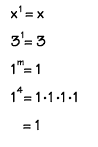Product Rule - The exponent "product rule" tells us that, when multiplying two powers that have the same base, you can add the exponents. In this example, you can see how it works. Adding the exponents is just a short cut!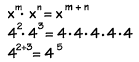Power Rule - To raise a power to a power, multiply the exponents. Here you see that 52 raised to the 3rd power is equal to 56.Quotient Rule -  We can divide two powers with the same base by subtracting the exponents. You can see why this works if you study the example shown.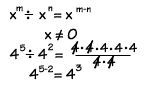Zero Rule - Any nonzero number raised to the power of zero equals 1.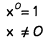Negative Exponents - Any nonzero number raised to a negative power equals its reciprocal raised to the opposite positive power.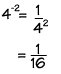Be careful when combining!
Terms like x2yz and xy2z look a lot alike, but they aren't and cannot be combined. Write the terms carefully when working out problems.

Don't overlook terms that are alike!
Terms obey the associative property of multiplication - that is, xy and yx are like terms, as are xy2 and y2x.

SIMPLIFYING -  Whenever a problem can be simplified, you should simplify it before substituting numbers for the letters. This will make your job a lot easier! To simplify an algebraic expression: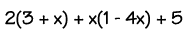1. Clear the parentheses.
2. Combine like terms by adding coefficients.
3. Combine the constants.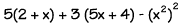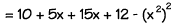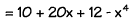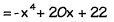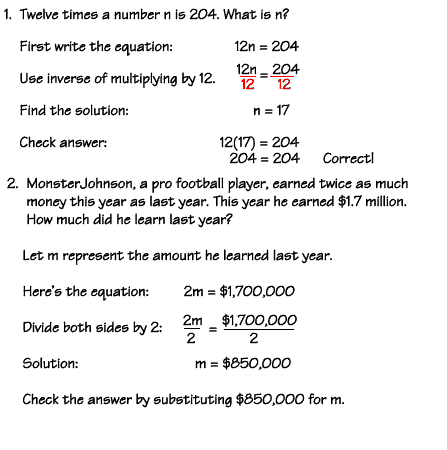The continental United States - the lower 48 states - has about 16,900 miles of shoreline. This is about ½ the length of the shoreline of Alaska. About how many miles of shoreline does Alaska have?

We can write a division equation to find the answer to problems like this. Our unknown number is the length of shoreline in Alaska. Let x represent this number. We know that x divided by 2 is the approximate length of shoreline in the lower 48 states. We can write the equation like this: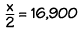To solve this equation, we can use the inverse of dividing by 2, or multiplying by 2. If we multiply the left side of the equation by 2, we will get x alone on the left. Remember, any operation done to one side must also be done to the other side, so we must also multiply the right side by 2.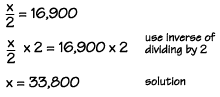We multiply, and find that x, the length of the shoreline of Alaska, is equal to 33,800 miles.

To check our answer, we substitute the value of 33,800 into the original equation, like this.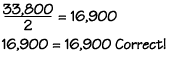F=9/5C+32  calculates farenheit to celsius

Distance = Rate x Time

********

x axis is on the horizontal

y axis is on the vertical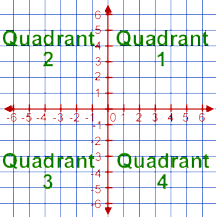The coordinate plane . Slope and y-intercept. Graphing linear equations.
Graphing Equations and Inequalities

The above section created with the assistance of math.com

Pythagorean Theorem:   The right triangle is a geometrical figure, used in many applications for thousands of years.  A Greek mathematician named Pythagoras developed the Pythagorean Theorem to help find the lengths of the sides of any right triangle.  Each side of a right triangle can be viewed as the side of a square, where the total area of the two smaller squares is equal to the area of the largest square: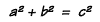where c is the hypotenuse and a and b are the other two legs of the triangle. Move your mouse over the triangle to learn more.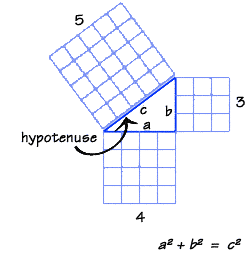A Three-Dimensional Tour Through Mathematics by Meer & Gardner

 For fun, colorful, interactive online tutorials:         http://emathonline.com/WMM/wmm.php   http://hotmath.com/math-tests.html   http://hotmath.com/help/videos/index.html   http://math.com/homeworkhelp/PreAlgebra.html   http://amby.com/educate/math/integer.html   (for signed numbers)  http://math.com/school/subject1/practice/S1U1L10/S1U1L10Pract.html     Four Unit Quizzes on this page:  http://math.com/practice/PreAlgebra.html   Click "Practice Subjects" for Pre-Algebra, Geometry & Algebra: http://math.com/students/practice.html   more math tests:  http://www.westcler.org/gh/outtda/practest.htm   Click on "Homework": http://hotmath.com/help/bookindexes/cpm02/index.html   college entrance exam tests: http://www.collegeboard.com/student/testing/ap/prep_free.html#music     ACT info, tips, and practice tests: http://actstudent.org/testprep/index.html   ModuMath videos from the library.   Review:   A parabola is the graph of a quadratic equation, such as:              y = ax2 + bx + c       or  y = a(x - h)2 + k.   Parabolas are concerned with the x-intercept, y-intercept, and the vertex.